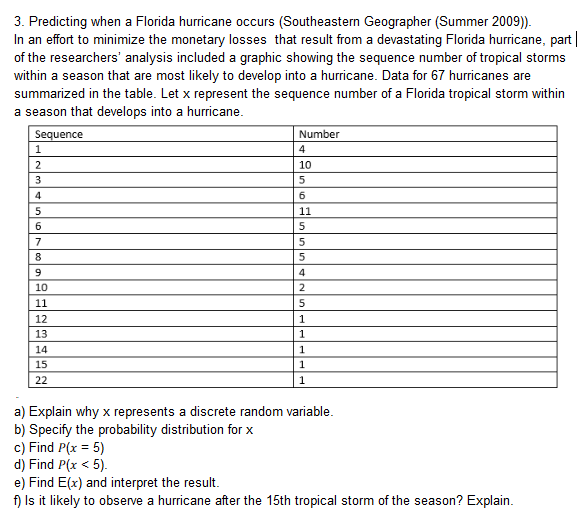# 3. Predicting when a Florida hurricane occurs (Southeastern Geographer (Summer 2009))In an effort to minimize the monetary losses that result from a devastating Florida hurricane, partof the researchers' analysis included a graphic showing the sequence number of tropical stormswithin a season that are most likely to develop into a hurricane. Data for 67 hurricanes aresummarized in the table. Let x represent the sequence number of a Florida tropical storm withina season that develops into a hurricaneenceNumber1012131415a) Explain why x represents a discrete random variableb) Specify the probability distribution for xc) Find P(x-5)d) Find P(x < 5)e) Find E(x) and interpret the resultf) Is it likely to observe a hurricane after the 15th tropical storm of the season? Explain

Question
530 views

Part d, e, fhelp_outlineImage Transcriptionclose3. Predicting when a Florida hurricane occurs (Southeastern Geographer (Summer 2009)) In an effort to minimize the monetary losses that result from a devastating Florida hurricane, part of the researchers' analysis included a graphic showing the sequence number of tropical storms within a season that are most likely to develop into a hurricane. Data for 67 hurricanes are summarized in the table. Let x represent the sequence number of a Florida tropical storm within a season that develops into a hurricane ence Number 10 12 13 14 15 a) Explain why x represents a discrete random variable b) Specify the probability distribution for x c) Find P(x-5) d) Find P(x < 5) e) Find E(x) and interpret the result f) Is it likely to observe a hurricane after the 15th tropical storm of the season? Explain fullscreen
check_circle

Step 1

The probability distribution for the variable x:

Conditions of the discrete probability distribution:

• The probabilities of all the values of the variable X must lie between 0 and 1.
• The sum of probabilities of all the values of X must be equal to “1”.

The total number of developed hurricanes is n = 67.

The general formula to obtain the probability of an event is,

Probability of an event = (Number of favourable cases for the event)/(Total number of cases).

Here, the probabilities for the values of the variable x is obtained as,

Probability of xi = (Number of hurricanes developed for that particular xi)/(Total number of hurricanes).

The probability distribution table of the variable x is given below:

Step 2

d.

Find the value of P(x < 5).

Here, the sequence number of tropical storms within a season is less than 5. That is, the variable x takes the values 1, 2, 3 and 4.

From the above obtained probability distribution of the variable x, the value of P(x = 1) is 0.0597, the value of P(x = 2) is 0.1493, the value of P(x = 3) is 0.0746, the value of P(x = 4) is 0.0896.

The value of P(x < 5) is obtained as 0.3732 from the calculation given below:

Step 3

e.

Find the mean of the variable x.

The general formula to obtain the expected value of a discrete random variable is,

E(X) = ∑X*P(X)

The values of x  and P(x) taken from the probability distribution table ob...

### Want to see the full answer?

See Solution

#### Want to see this answer and more?

Solutions are written by subject experts who are available 24/7. Questions are typically answered within 1 hour.*

See Solution
*Response times may vary by subject and question.
Tagged in

### Other